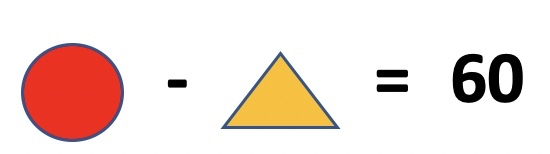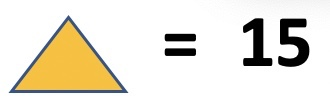#### Olympiad Math Preparation – Class 2 – Computation Operations – Subtraction of 2 and 3 digit numbers ( with regrouping/borrow

##### 1. Which number is less than 400 by 82 ?

2. What is difference between the greatest and smallest numbers ?

Numbers are : 167, 456, 389, 345 , 212

3. The difference between smallest three digit number and greatest one digit number is ______________

4. The difference between number shown in abacus is _____________________________

5. Complete the series : 200 , 180 , 160 , 140 , ________

6. What is difference between 10 tens and 6 ones

7. How many tens are there in difference between numbers : 679 and 479 ?

### Olympiad Math Preparation – Class 2 -Subtraction of 2 and 3 digit numbers ( without regrouping/borrow )

1. The difference between greatest and smallest number is _________________ . Numbers : 178, 608, 578, 801, 999

2. Select the correct option

3. The difference of numbers shown in two abacuses below is ________________

4. Ifandthen what is value of?

5. Ifis subtracted from 100, the result is 45. Thenis _________.

6. What is difference of largest and smallest 3 digit number ?

7. Duggu has 85 chocolates. Dakshu has 24 chocolates. How many more chocolates Duggu has than Dakshu ?

8.and. Find the value ofRELATED POSTS :

# Olympiad Math Preparation – Class 2

## Addition of 2 and 3 digit numbers ( without regrouping/carry )

1. The sum of greatest and smallest number is _________________ . Numbers : 188, 508, 345, 801, 290

2. Select the correct option

3. The sum of numbers shown in two abacuses below is ________________

4. Ifandthen what is value of?

5. Ifis added to 100, the result is 150. Thenis _________.

6. What is sum of largest and smallest 3 digit number ?

7. Duggu has 54 chocolates. Dakshu has 45 chocolates. How many chocolates both of them have ?

8.and. Find the value of